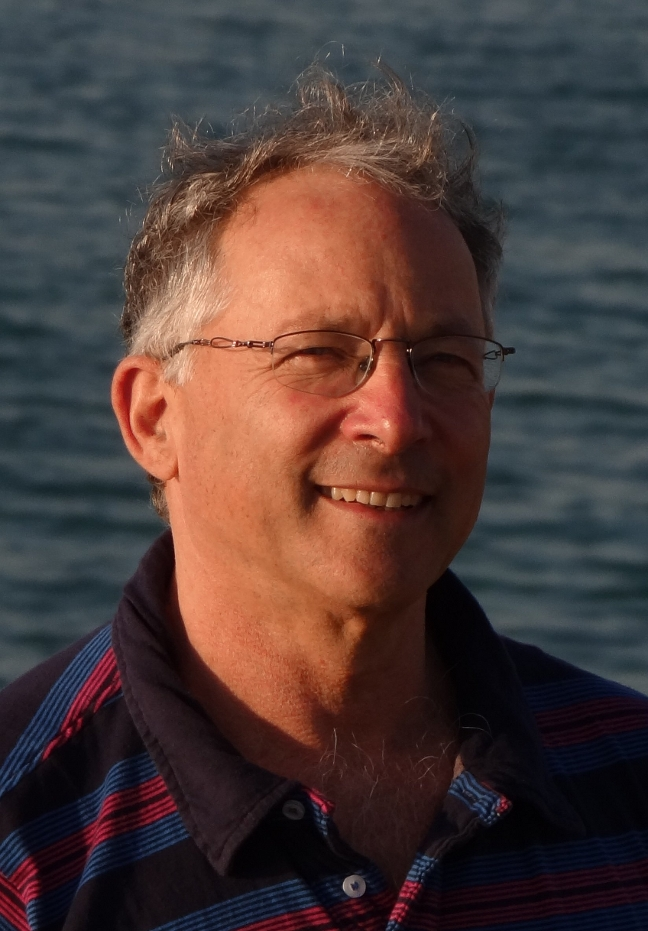# Shai Ben-David

Professor

School of Computer Science
Universitys of Waterloo
200 University Avenue West
Waterloo, Ontario, Canada, N2L 3G1

Email: "MyFirstName"@cs.uwaterloo.ca
Phone: +1 519 888 4567 ext. 37523
Fax: +1 519 885-1208

### Alternative Measures of Complexity

A fundamental problem of complexity theory: Worst-case complexity is the most common and researched measure of computational complexity. However, it is known to give rise to over-pessimistic complexity results. Many problems that are hard from the point of view of that theory turn out to be efficiently solvable in practice (SAT is the most conspicuous of those). A variety of algorithmic problems in machine learning fall in that category. In (TAMC 06), I propose an alternative measure of computational complexity that takes into account not just the input size but also its "noise robustness" w.r.t. a given task. The new measure coincides with the common worst-case complexity for "nicely behaving" inputs, but becomes less demanding for noise-sensitive inputs. I introduced algorithms that are computationally efficient w.r.t. the new measure for a variety of NP-hard tasks (MLJ 2007, NIPS2000, AISTATS 2009). Assuming that inputs that arise naturally in machine learning are nicely behaved, these results provide a possible explanation to the above mentioned theory-practice gap.

However, the problem is still wide open: The algorithms described above are not practical, and the scope of problems they apply to is only a small fraction of the wide range of problems demonstrating the practice-theory gap described above. This is a very challenging and exiting area of research that is currently addressed by several groups around the word.